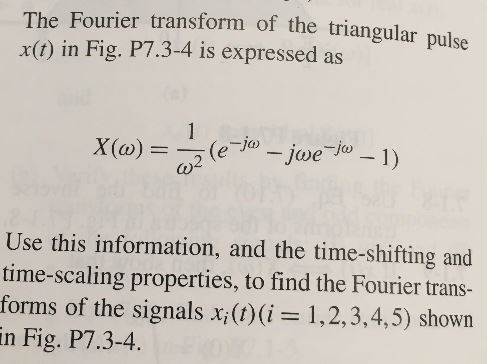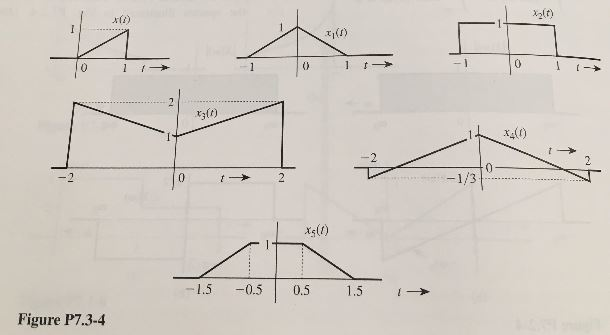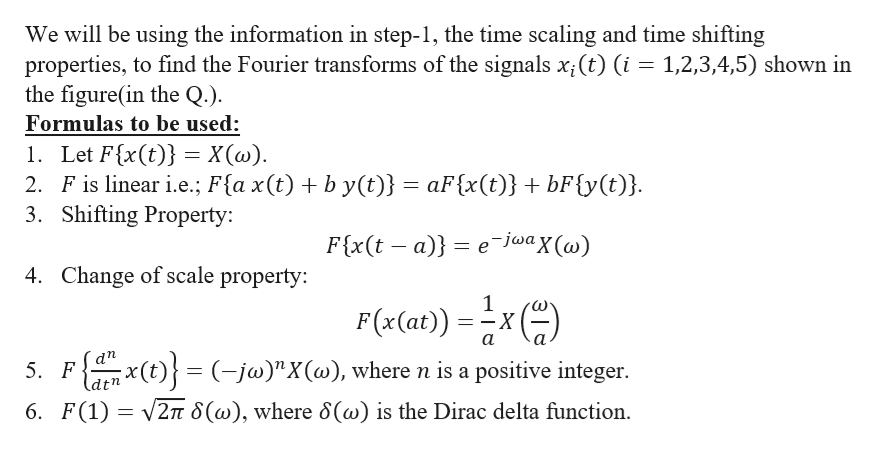# The Fourier transform of the triangular pulsex(t) in Fig. P7.3-4 is expressed as1X(a)(e Jjwe Jo-1)Use this information, and the time-shifting andtime-scaling properties, to find the Fourier trans-forms of the signals x;(t)(i= 1,2,3,4,5) shownin Fig. P7.3-4 - 1012-1/3-1.5-0.50.51.5Figure P7.3-4

Question
46 views

Determine a matrix representation if possible for each mapping in the problem.help_outlineImage TranscriptioncloseThe Fourier transform of the triangular pulse x(t) in Fig. P7.3-4 is expressed as 1 X(a) (e Jjwe Jo-1) Use this information, and the time-shifting and time-scaling properties, to find the Fourier trans- forms of the signals x;(t)(i= 1,2,3,4,5) shown in Fig. P7.3-4 fullscreenhelp_outlineImage Transcriptionclose- 1 0 1 2 -1/3 -1.5 -0.5 0.5 1.5 Figure P7.3-4 fullscreen
check_circle

Step 1

As per our norms, we will be answering only first 3 sub-parts. If you would like to get the answers of other sub-parts, then kindly re-post the same specifying the required sub-parts.

Step 2

We are given the Fourier transform of the triangular pulse x(t) of the figure below is expressed as

Step 3

Here,

...help_outlineImage TranscriptioncloseWe will be using the information in step-1, the time scaling and time shifting properties, to find the Fourier transforms of the signals x(t) (i 1,2,3,4,5) shown in the figure(in the Q.) Formulas to be used: 1. Let F{x(t) = X(w) 2. F is linear i.e.; F{a x(t) b y(t)} aF{x(t)} + bF{y(t)}. 3. Shifting Property: e-jwax (w) F{x(t - a) 4. Change of scale property: 1 F(x(at)) X а аn (t(-jw)"X(w), where n is a positive integer 5. F dtn 6. F(1)2 8(w), where 8(@) is the Dirac delta function fullscreen

### Want to see the full answer?

See Solution

#### Want to see this answer and more?

Solutions are written by subject experts who are available 24/7. Questions are typically answered within 1 hour.*

See Solution
*Response times may vary by subject and question.
Tagged in

### Math